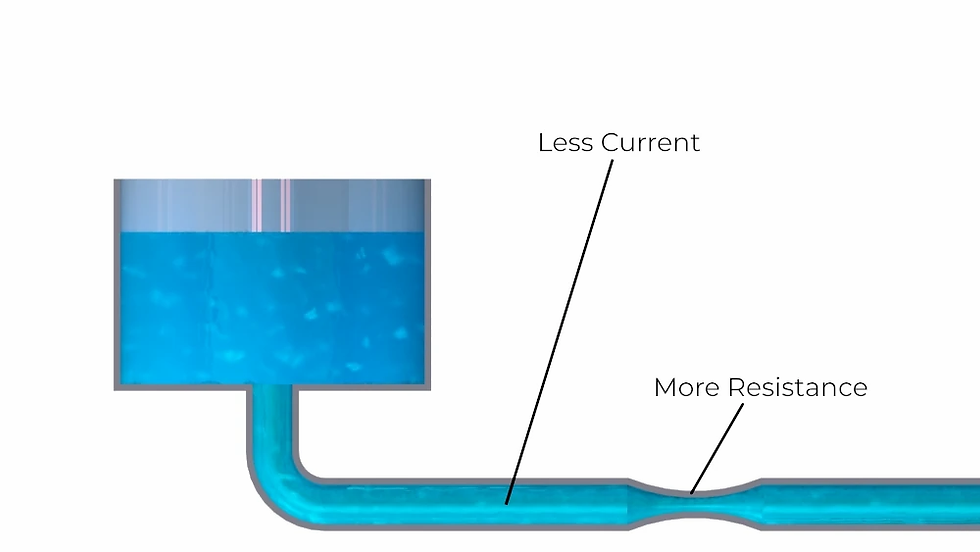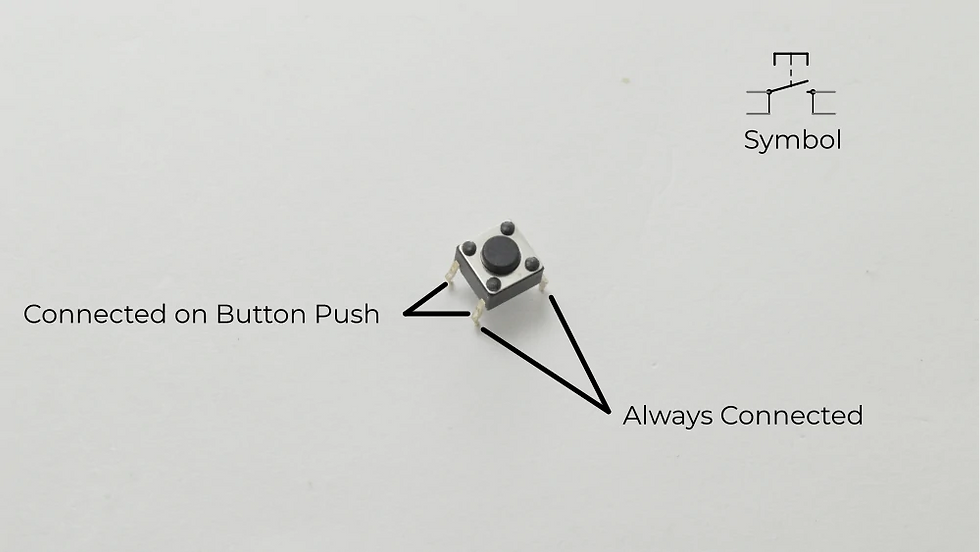top of page
Search

# Building a Circuit on a Breadboard

Updated: Jan 2, 2022

Before diving into some programming with the Arduino Uno and the IDE, it's important to know some basic circuit theory and how to build these circuits on a breadboard.

In this post, I will discuss:

• the basics of electrical circuits

• three basic examples related to a series and parallel circuits

Below is a list parts that are used in the examples from this post. All of these parts can be found in the Arduino Starter Kit.

Basic Circuit Theory

It's important to understand the concept of voltage, resistance, and current. Voltage, measured in Volts (V), is the difference of electrical charge between two points of the circuit. Resistance, measured in Ohms (Ω), is a measure of the resistance to the flow of electrical energy. Current, measured in amperes, or amps (A), is the measure of how much electrical charge is passing by a given point in the circuit.

With electrical circuits, I like to use water flowing through a pipe as an analogy. Imagine an elevated water tank connected to a pipe with a small section of the pipe that is narrow. The height of the water level can be thought of as voltage. The flow of water is analogous to current, and the narrowing of the pipe is similar to resistance of an electric circuit.Electrical Circuit Water Analogy

Voltage, current, and resistance are related to formula called Ohm’s Law. This formula states that the voltage of a circuit is equal to the current times the resistance (V = IR). From this formula you can see that if you increase the resistance, you decrease the current (i.e. narrowing the pipe increases resistance and reduces current).A Smaller Pipe Reduces Current

It can also be noted that an increase in voltage will increase the current (i.e. a higher water level increases current).A Higher Water Level Increases Current

Keep this water analogy in mind when you’re trying to think about what’s happening with your circuit. It's important to understand Ohm's Law and current, because the Arduino Uno has a limit to how much current flows through it. If the current gets too high, the board can be damaged.

Electrical Components

Resistors are the components that resist the flow of current. They have resistance values that are measured in ohms, represented by the Greek letter Omega (Ω). They have different color coding that varies depending on the resistance values. A great resource to determine the resistance values can be found here.Colored Bands on Resistors

Being colorblind, I find that the easiest way for me to determine resistance values is by using a multi-meter.Measuring Resistance with a Multimeter

An LED, or a light emitting diode, is a component that emits light when a voltage is applied to it. LED’s only allow the current to flow in one direction. The two leads of the LED are called the anode and cathode. The longer lead is the anode and is connected to positive. The shorter lead is the cathode and is connected to negative.Light Emitting Diode (LED)

Switches are components that break the flow of electricity. The switches in the starter kit are push buttons, meaning the circuit is only closed if the button is being pushed. The two leads that are attached to the same side are connected when the buttons is being pressed down. The two leads that are on opposite sides are always connected, essentially acting like a wire.Push Button

The breadboard is the component that allows you to create circuits without having to solder wires together. On the breadboard, the vertical columns on the sides are connected by conductive strips, commonly known as the power buses. The horizontal rows are also connected. The connections between the two sides of the breadboard are broken by a gap.Example 1: Simple Circuit

This first example involves simply powering an LED using the 5V output from the Arduino.

• First, connect 5V and ground to the power bus rails on the breadboard.

• Then connect one end of a 220Ω resistor to the positive power rail, and the other end to one of the leads of the push button.

• Connect the other end of the push button to the anode of the LED.

• Connect cathode of the LED to ground.Simple Circuit Diagram

What should happen is that the LED will turn on when the button is pushed and turn off with it is released. If the LED doesn't turn on, the polarity is likely reversed. Turn the LED around on the breadboard to solve this problem. All LED’s need to be wired to a resistor. It doesn’t matter whether it is connected to the anode or cathode.Simple LED Circuit

Example 2: Series Circuit

The Second example will demonstrate a series circuit with two LED's and a single push button.

• Just like before, connect one end of a 220Ω resistor to the positive rail.

• Wire the other end of the resistor to one end of the push button.

• Connect the other end of the button to the anode of an LED.

• Connect the cathode of that same LED to the anode of another LED.

• Then connect the cathode of the second LED to ground.Series Circuit Diagram

In this case, both LED’s will be activated simultaneously when the button is pushed. This is because the LED’s are wired in series.LED's in Series

Example 3: Parallel Circuit

The last example demonstrates a parallel circuit with two LED’s.

• Start by connecting 5V and ground to the power bus.

• Connect one end of a 220Ω resistor to the positive rail.

• Connect the other end of the resistor to one of the leads of a push button.

• Wire the other side of the push button to the anode of an LED.

• Wire the cathode of the same LED to ground.

• Next, create the same circuit in a different location on the breadboard so that the switch is connected to the same resistor.

• Ground the cathode of the other LED.Parallel Circuit Diagram

In this case, individual LED’s will only turn on if the corresponding push button is pressed. Both lights will only go on if both push buttons are pressed.Parallel LED Circuit with One Light Turned OnParallel LED Circuit with One Light Turned OnParallel LED Circuit with Both Lights On

In the upcoming posts in this series, we'll start getting into programming and how to use more components in the kit.

If you would like to stay up to date with this Introduction to Arduino series, subscribe below.

The Bored Robot LLC is a participant in the Amazon Services LLC Associates Program, an affiliate advertising program designed to provide a means for sites to earn advertising fees by advertising and linking to Amazon.com